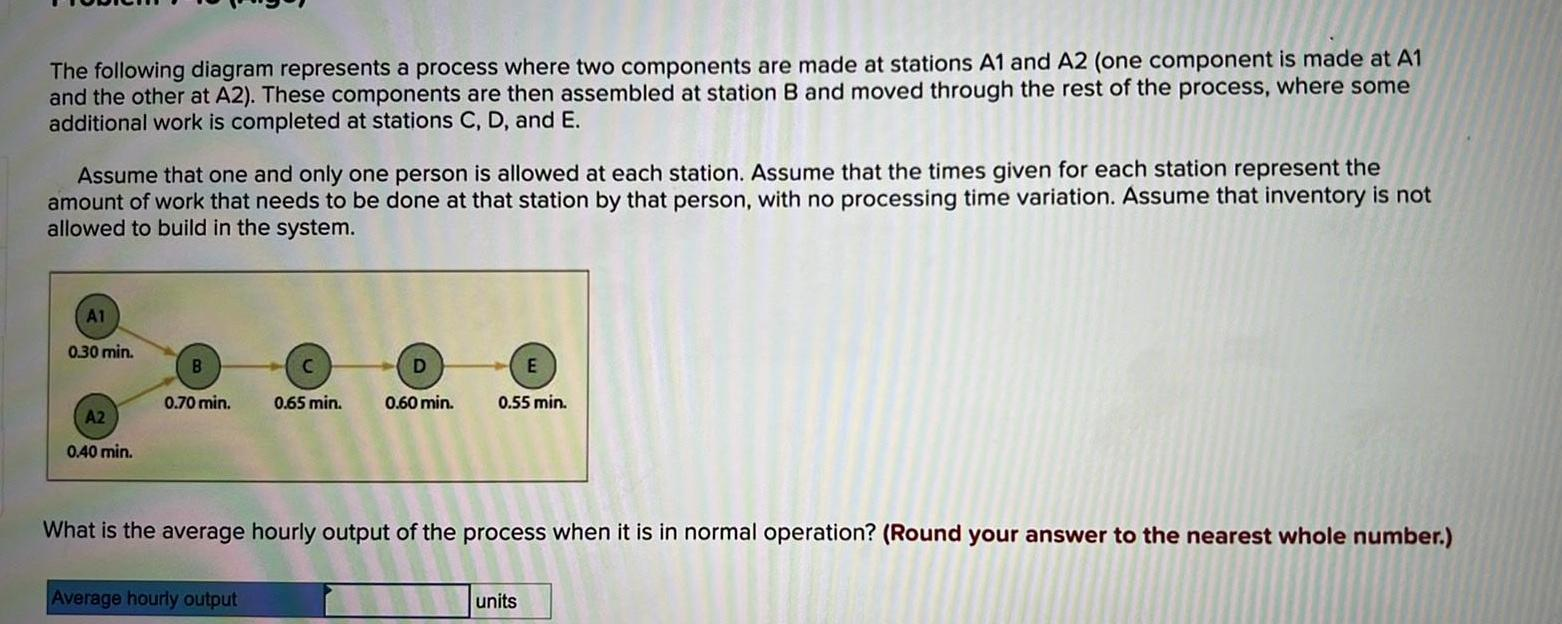Question:

# The following diagram represents a process where two

Last updated: 9/15/2023The following diagram represents a process where two components are made at stations A1 and A2 one component is made at A1 and the other at A2 These components are then assembled at station B and moved through the rest of the process where some additional work is completed at stations C D and E Assume that one and only one person is allowed at each station Assume that the times given for each station represent the amount of work that needs to be done at that station by that person with no processing time variation Assume that inventory is not allowed to build in the system A1 0 30 min A2 0 40 min B 0 70 min D 0 65 min 0 60 min Average hourly output E 0 55 min What is the average hourly output of the process when it is in normal operation Round your answer to the nearest whole number units